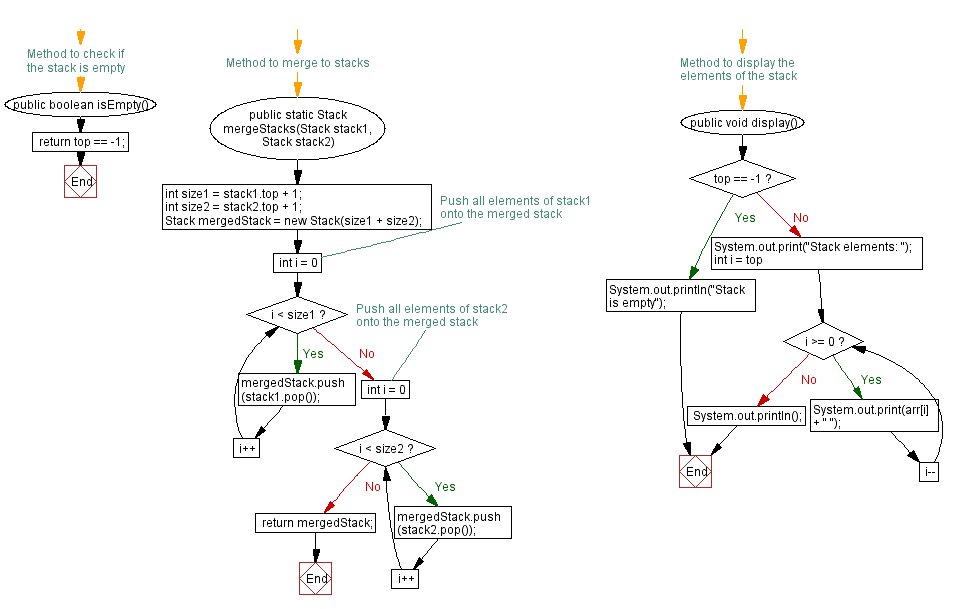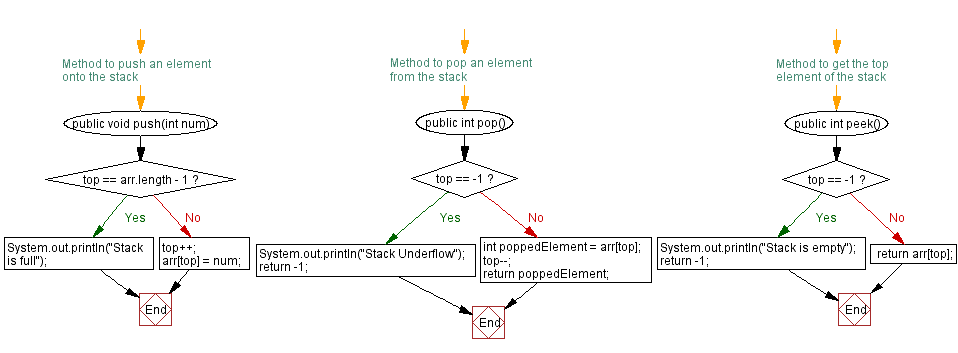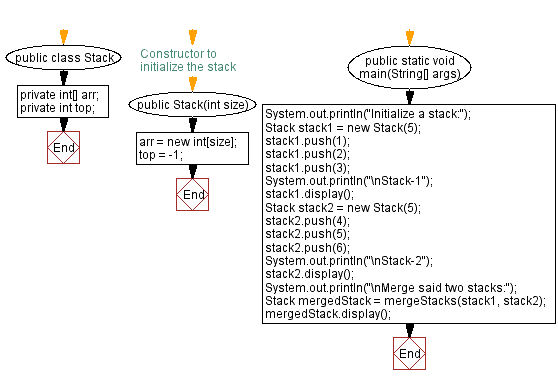# Java Exercises: Merge two stacks into one

## Java Stack: Exercise-18 with Solution

Write a Java program to merge two stacks into one.

Sample Solution:

Java Code:

``````import java.util.Scanner;

public class Stack {
private int[] arr;
private int top;

// Constructor to initialize the stack
public Stack(int size) {
arr = new int[size];
top = -1;
}

// Method to push an element onto the stack
public void push(int num) {
if (top == arr.length - 1) {
System.out.println("Stack is full");
} else {
top++;
arr[top] = num;
}
}

// Method to pop an element from the stack
public int pop() {
if (top == -1) {
System.out.println("Stack Underflow");
return -1;
} else {
int poppedElement = arr[top];
top--;
return poppedElement;
}
}

// Method to get the top element of the stack
public int peek() {
if (top == -1) {
System.out.println("Stack is empty");
return -1;
} else {
return arr[top];
}
}

// Method to check if the stack is empty
public boolean isEmpty() {
}
// Method to merge to stacks
public static Stack mergeStacks(Stack stack1, Stack stack2) {
int size1 = stack1.top + 1;
int size2 = stack2.top + 1;
Stack mergedStack = new Stack(size1 + size2);

// Push all elements of stack1 onto the merged stack
for (int i = 0; i < size1; i++) {
mergedStack.push(stack1.pop());
}

// Push all elements of stack2 onto the merged stack
for (int i = 0; i < size2; i++) {
mergedStack.push(stack2.pop());
}

return mergedStack;
}

// Method to display the elements of the stack
public void display() {
if (top == -1) {
System.out.println("Stack is empty");
} else {
System.out.print("Stack elements: ");
for (int i = top; i >= 0; i--) {
System.out.print(arr[i] + " ");
}
System.out.println();
}
}

public static void main(String[] args) {
System.out.println("Initialize a stack:");
Stack stack1 = new Stack(5);
stack1.push(1);
stack1.push(2);
stack1.push(3);
System.out.println("\nStack-1");
stack1.display();
Stack stack2 = new Stack(5);
stack2.push(4);
stack2.push(5);
stack2.push(6);
System.out.println("\nStack-2");
stack2.display();
System.out.println("\nMerge said two stacks:");
Stack mergedStack = mergeStacks(stack1, stack2);
mergedStack.display();

}
}
```
```

Sample Output:

```Stack-1
Stack elements: 3 2 1

Stack-2
Stack elements: 6 5 4

Merge said two stacks:
Stack elements: 4 5 6 1 2 3

```

Flowchart:Live Demo:

Java Code Editor:

Improve this sample solution and post your code through Disqus

Java Stack Previous: Move the nth element from the top of the stack to the top.
Java Stack Exercises Next: Check if the stack is a subset of another stack.

What is the difficulty level of this exercise?

Test your Programming skills with w3resource's quiz.

﻿